# Butler Matrix

The Butler matrix is a type of beam-forming network. Depending on which of N inputs is accessed, the antenna beam is steered in a specific direction in one plane; Butler matrices can be combined in two "layers" to facilitate 3D scanning. It performs a similar function to a Rotman lens, or a phased array antenna system.

The Butler matrix was first described by Jesse Butler and Ralph Lowe in a paper titled Beam-Forming Matrix Simplifies Design of Electronically Scanned Antennas, Electronic Design, volume 9, pp. 170-173, April 12, 1961. Butler was an employee of Sanders Associates in Nashua, New Hampshire, which is now part of BAE. It is remarkable that the chose not to first describe what they called the "Sanders beam-forming matrix" in an IEEE journal.

The primary characteristics of the Butler matrix are:

1. N inputs and N outputs, with N usually 4, 8 or 16
2. Inputs are isolated from each other
3. Phases of N outputs are linear with respect to position, so beam is tilted off main axis.
4. None of the inputs provides a broadside beam
5. The phase increment between the outputs depends on which input you use

By the way, this is a passive reciprocal network, so it works the same when it transmits energy as when it receives energy, but in our analysis we'll assume that it is employed as a transmitter, with the inputs on the left and the antenna elements on the right.

The schematic below is for a simple, ideal Butler matrix that will drive four elements. Quadrature couplers are needed in four places, we chose to use the branchline coupler; it is comprised of four lines forming a box, with the vertical elements at impedance equal to Z0, and the horizontal elements at Z0/[sqrt(2)]. Two 45 degree fixed phase shifters are needed, here these are series lines sized to provide 45 degrees in length. Note that two crossovers are required, so the Butler matrix requires some type of 3D interconnect.

.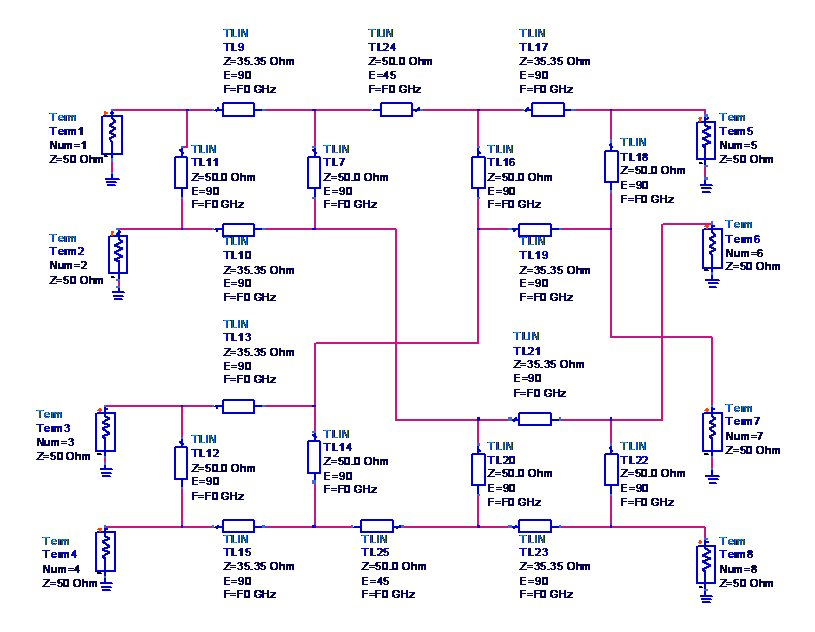We chose the center frequency of of our ideal network to be 10 GHz. Below phase responses between four inputs and four outputs are plotted on Smith charts over very small frequency range. It may be difficult to see, but when port 1 is the input, the four outputs are linearly phased in 45 degree increments (port 5 is at zero phase angle). When port 2 is the input, the phase increment is now 135 degrees, with port 5 now at 90 degrees. When port 3 is the input, the phase increment is 270 degrees (port 5 is at 45 degrees). When port 4 is the input, the outputs are phased at 315 degree increments (port 5 is now at 135 degrees).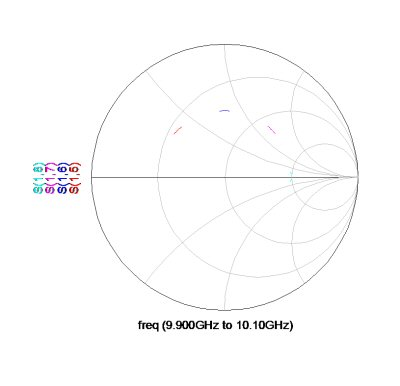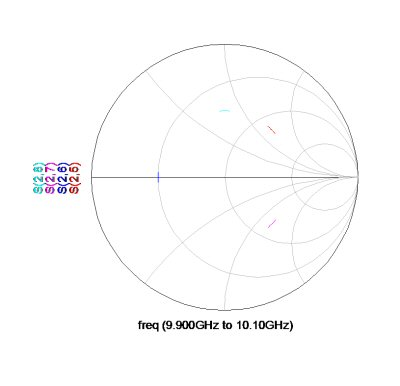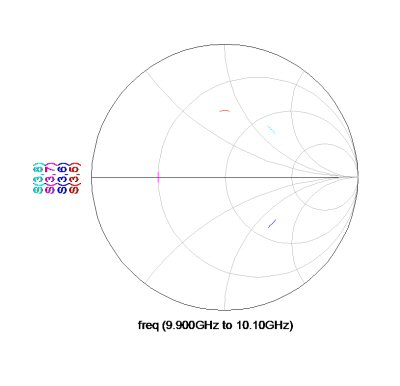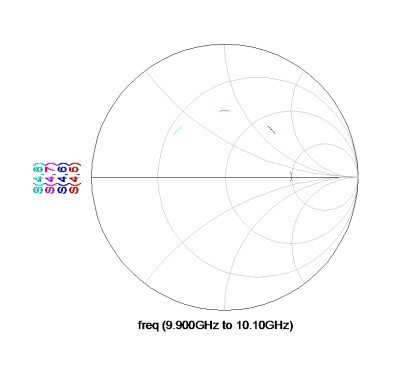The magnitudes of the responses from the four inputs to four outputs are plotted below, over 8 to 12 GHz. Note that the usable response is perhaps less than one GHz.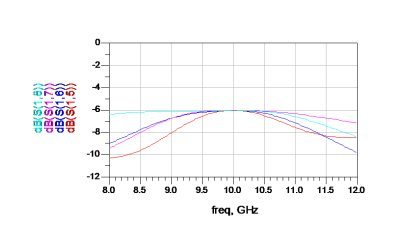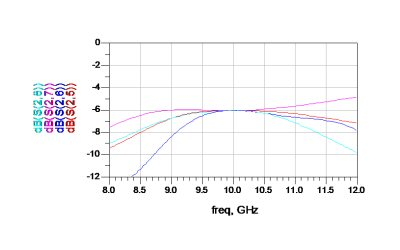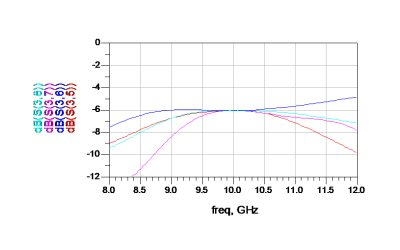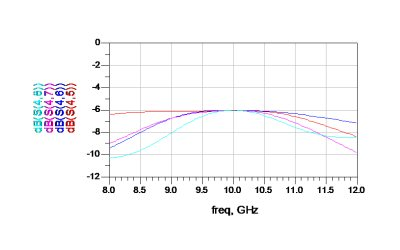To improve the bandwidth of the Butler matrix, there are two things you must do. First, you need to replace the branchline couplers with couplers that have a "natural" quadrature coupling, such as the Lange, or other coupled line coupler, or in the case of waveguide, the Riblett coupler. You should also consider replacing the 45 degree phase-shifting lines with Schiffman phase shifters (for flatter phase response over frequency).

One of these days we'll put together an Excel spreadsheet that plots the array factor of this example!

Author : Unknown Editor